## Exploratory analysis

download.file("http://www.openintro.org/stat/data/nc.RData", destfile = "nc.RData")
load("nc.RData")

### What are the cases in this data set? How many cases are there in our sample?

#### The cases are Babies born in North carolina, and ther are 100 cases in the sample

nrow(nc)
##  1000
names(nc)
##   "fage"           "mage"           "mature"         "weeks"
##   "premie"         "visits"         "marital"        "gained"
##   "weight"         "lowbirthweight" "gender"         "habit"
##  "whitemom"
head(nc)
##   fage mage      mature weeks    premie visits marital gained weight
## 1   NA   13 younger mom    39 full term     10 married     38   7.63
## 2   NA   14 younger mom    42 full term     15 married     20   7.88
## 3   19   15 younger mom    37 full term     11 married     38   6.63
## 4   21   15 younger mom    41 full term      6 married     34   8.00
## 5   NA   15 younger mom    39 full term      9 married     27   6.38
## 6   NA   15 younger mom    38 full term     19 married     22   5.38
##   lowbirthweight gender     habit  whitemom
## 1        not low   male nonsmoker not white
## 2        not low   male nonsmoker not white
## 3        not low female nonsmoker     white
## 4        not low   male nonsmoker     white
## 5        not low female nonsmoker not white
## 6            low   male nonsmoker not white
summary(nc)
##       fage            mage            mature        weeks
##  Min.   :14.00   Min.   :13   mature mom :133   Min.   :20.00
##  1st Qu.:25.00   1st Qu.:22   younger mom:867   1st Qu.:37.00
##  Median :30.00   Median :27                     Median :39.00
##  Mean   :30.26   Mean   :27                     Mean   :38.33
##  3rd Qu.:35.00   3rd Qu.:32                     3rd Qu.:40.00
##  Max.   :55.00   Max.   :50                     Max.   :45.00
##  NA's   :171                                    NA's   :2
##        premie        visits            marital        gained
##  full term:846   Min.   : 0.0   married    :386   Min.   : 0.00
##  premie   :152   1st Qu.:10.0   not married:613   1st Qu.:20.00
##  NA's     :  2   Median :12.0   NA's       :  1   Median :30.00
##                  Mean   :12.1                     Mean   :30.33
##                  3rd Qu.:15.0                     3rd Qu.:38.00
##                  Max.   :30.0                     Max.   :85.00
##                  NA's   :9                        NA's   :27
##      weight       lowbirthweight    gender          habit
##  Min.   : 1.000   low    :111    female:503   nonsmoker:873
##  1st Qu.: 6.380   not low:889    male  :497   smoker   :126
##  Median : 7.310                               NA's     :  1
##  Mean   : 7.101
##  3rd Qu.: 8.060
##  Max.   :11.750
##
##       whitemom
##  not white:284
##  white    :714
##  NA's     :  2
##
##
##
## 

### Exercise 2. Make a side-by-side boxplot of habit and weight. What does the plot highlight about the relationship between these two variables?

boxplot(weight~habit,data=nc, main="relationship between mother's habit and baby weight", ylab = "baby weight", xlab = "mother's habit")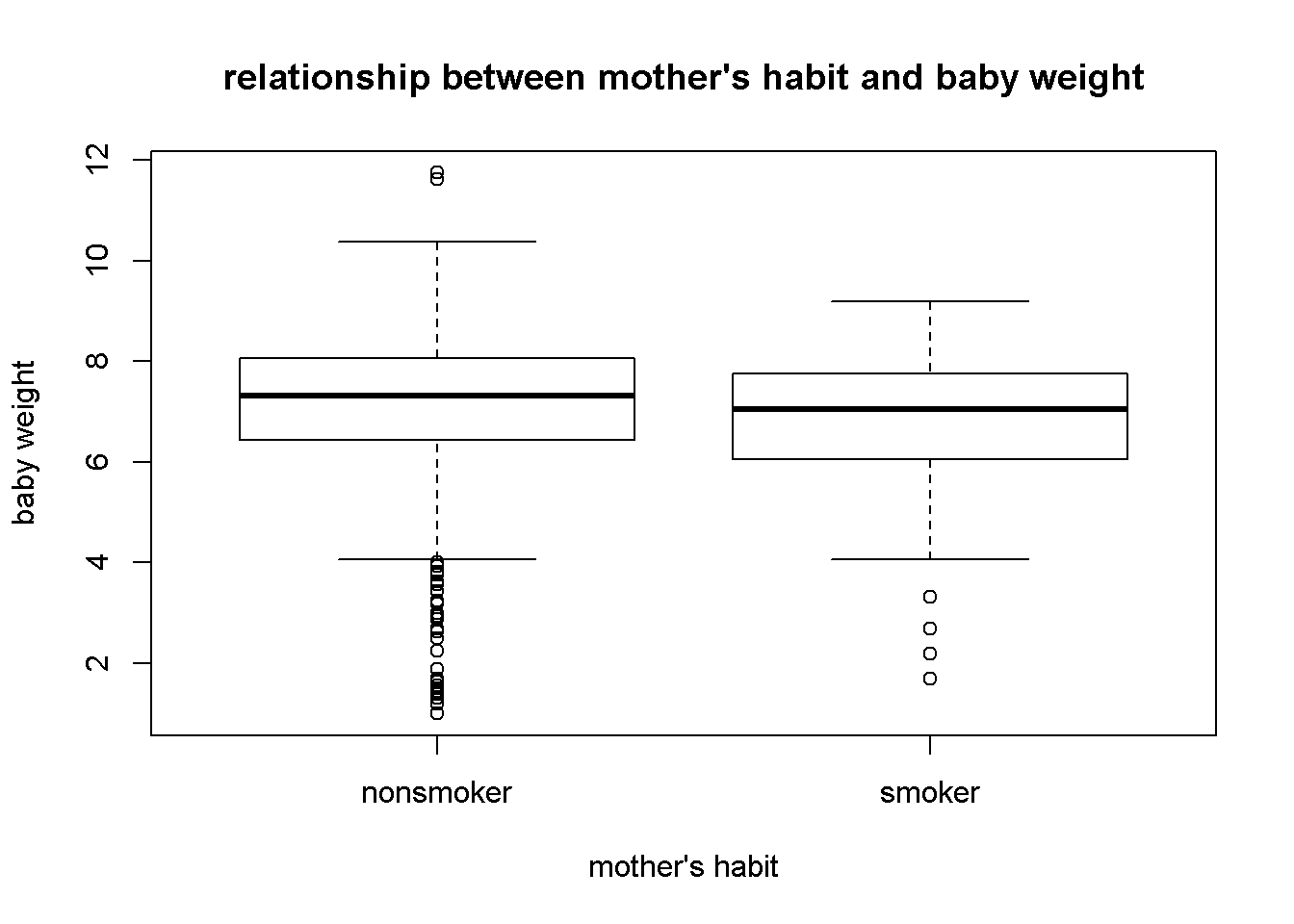#### Mothers who smoke gave birth to lighter baby in terms of weight compared to that of mothers who do not smoke

by(nc$weight, nc$habit, mean)
## nc$habit: nonsmoker ##  7.144273 ## -------------------------------------------------------- ## nc$habit: smoker
##  6.82873

##Inference ### Exercise 3. Check if the conditions necessary for inference are satisfied. Note that you will need to obtain sample sizes to check the conditions. You can compute the group size using the same by command above but replacing mean with length. #### Samples are independent and not bias and sample size is sufficiently large (100>30)

### Exercise 4. Write the hypotheses for testing if the average weights of babies born to smoking and non-smoking mothers are different.

#### The null hypothesis is there is no difference between the weight of babies between smoking and non-smoking mothers. Alternative hypothesis is there is a difference between weight of babies between smoking and nonsmoking mothers

inference(y = nc$weight, x = nc$habit, est = "mean", type = "ht", null = 0,
alternative = "twosided", method = "theoretical")
## Response variable: numerical, Explanatory variable: categorical
## Difference between two means
## Summary statistics:
## n_nonsmoker = 873, mean_nonsmoker = 7.1443, sd_nonsmoker = 1.5187
## n_smoker = 126, mean_smoker = 6.8287, sd_smoker = 1.3862
## Observed difference between means (nonsmoker-smoker) = 0.3155
##
## H0: mu_nonsmoker - mu_smoker = 0
## HA: mu_nonsmoker - mu_smoker != 0
## Standard error = 0.134
## Test statistic: Z =  2.359
## p-value =  0.0184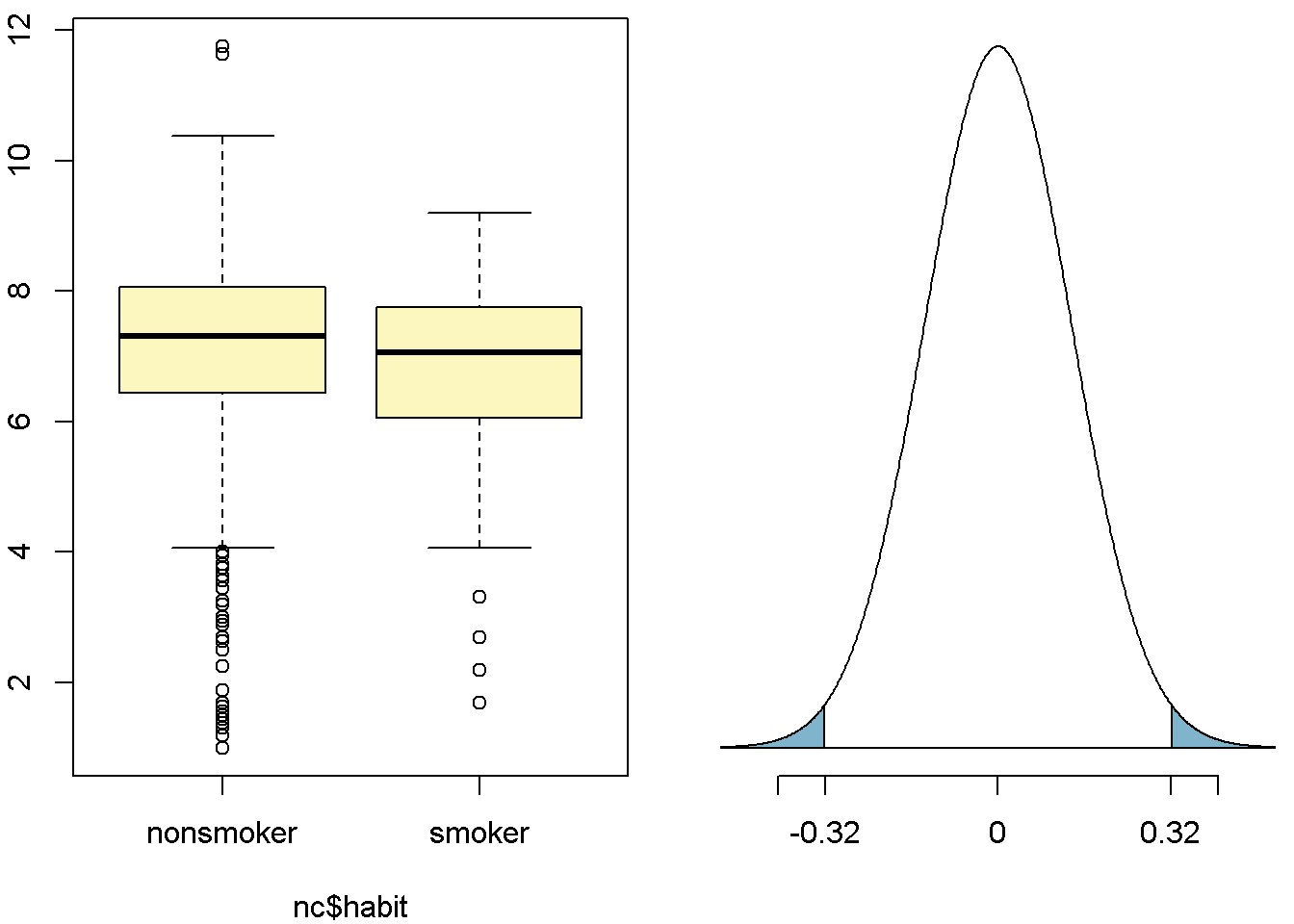### Exercise 5. Change the type argument to “ci” to construct and record a confidence interval for the difference between the weights of babies born to smoking and non-smoking mothers.

inference(y = nc$weight, x = nc$habit, est = "mean", type = "ci", null = 0,
alternative = "twosided", method = "theoretical")
## Response variable: numerical, Explanatory variable: categorical
## Difference between two means
## Summary statistics:
## n_nonsmoker = 873, mean_nonsmoker = 7.1443, sd_nonsmoker = 1.5187
## n_smoker = 126, mean_smoker = 6.8287, sd_smoker = 1.3862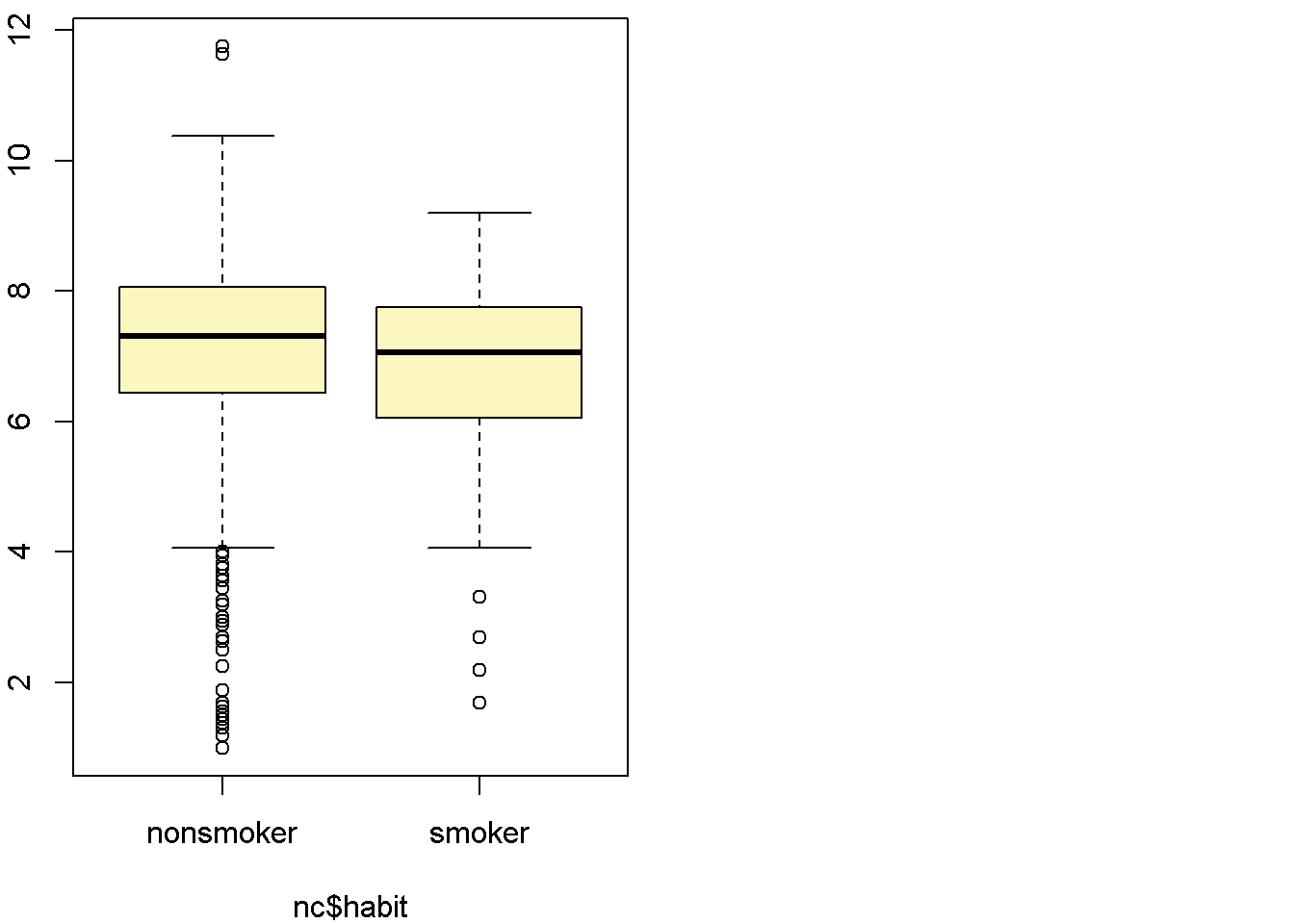## Observed difference between means (nonsmoker-smoker) = 0.3155
##
## Standard error = 0.1338
## 95 % Confidence interval = ( 0.0534 , 0.5777 )
inference(y = nc$weight, x = nc$habit, est = "mean", type = "ci", null = 0,
alternative = "twosided", method = "theoretical",
order = c("smoker","nonsmoker"))
## Response variable: numerical, Explanatory variable: categorical
## Difference between two means
## Summary statistics:
## n_smoker = 126, mean_smoker = 6.8287, sd_smoker = 1.3862
## n_nonsmoker = 873, mean_nonsmoker = 7.1443, sd_nonsmoker = 1.5187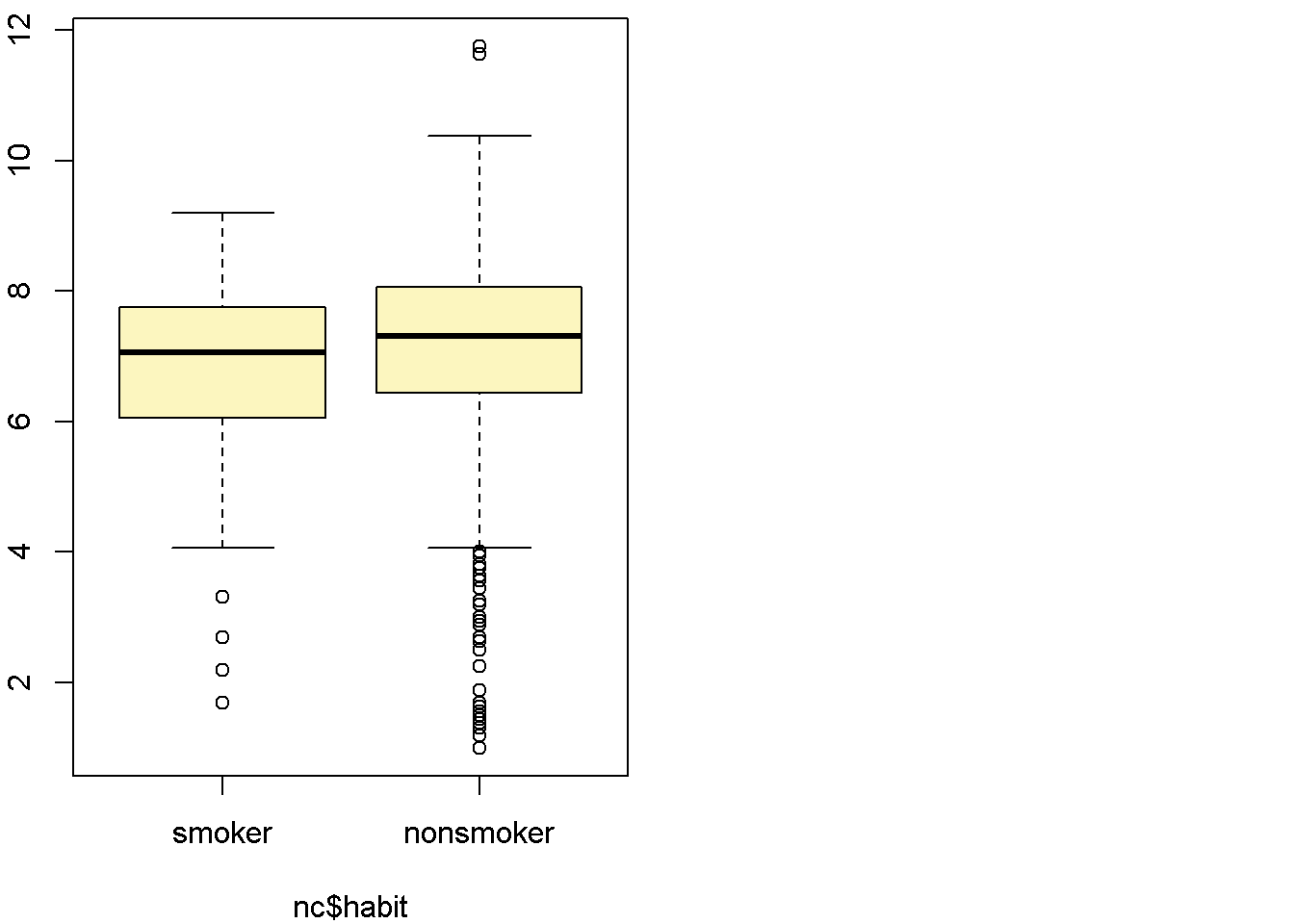## Observed difference between means (smoker-nonsmoker) = -0.3155
##
## Standard error = 0.1338
## 95 % Confidence interval = ( -0.5777 , -0.0534 )

##On your own ###1.Calculate a 95% confidence interval for the average length of pregnancies (weeks) and interpret it in context. Note that since you’re doing inference on a single population parameter, there is no explanatory variable, so you can omit the x variable from the function.

inference(y = nc$weeks, est = "mean", type = "ci", method = "theoretical") ## Single mean ## Summary statistics: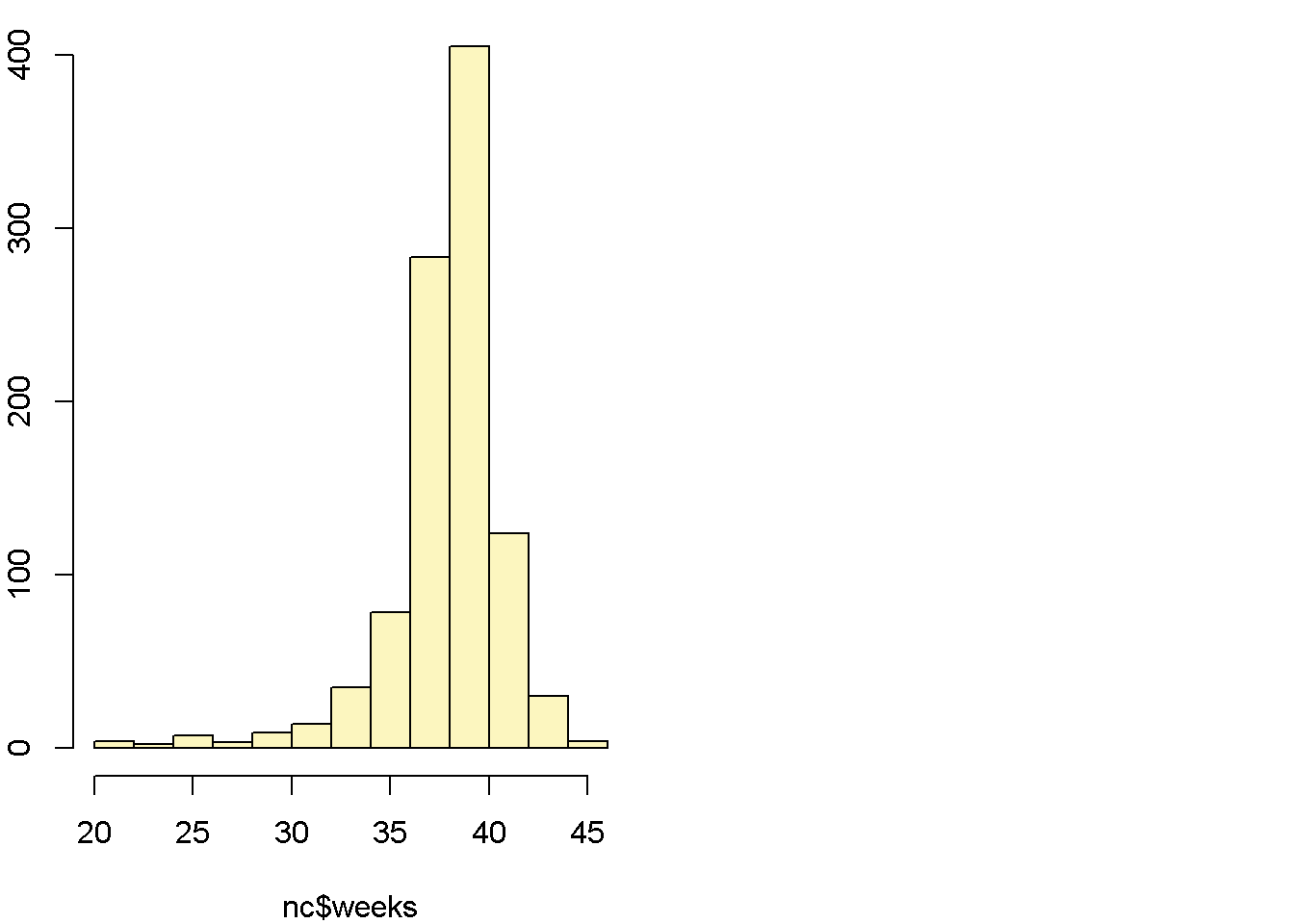## mean = 38.3347 ; sd = 2.9316 ; n = 998 ## Standard error = 0.0928 ## 95 % Confidence interval = ( 38.1528 , 38.5165 ) #### We are 95% confident that the true population mean for weeks of pregnancy before birth is between 38.15 weeks and 38.517 weeks. ###2.Calculate a new confidence interval for the same parameter at the 90% confidence level. You can change the confidence level by adding a new argument to the function: conflevel = 0.90. inference(y = nc$weeks, est = "mean", conflevel = 0.9, type = "ci", method = "theoretical")
## Single mean
## Summary statistics:## mean = 38.3347 ;  sd = 2.9316 ;  n = 998
## Standard error = 0.0928
## 90 % Confidence interval = ( 38.182 , 38.4873 )

#### The 90 % Confidence interval = ( 38.182 , 38.4873 )

###3.Conduct a hypothesis test evaluating whether the average weight gained by younger mothers is different than the average weight gained by mature mothers. #### Null hypothesis: There is no difference in weight gained during pregnancy between younger mothers and mature mothes. Alternative hypothesis: There is a difference in weight gained during pregnancy between younger mothers and mature mothes

inference(y = nc$weight, x = nc$mature, est = "mean", type = "ci", null = 0,
alternative = "twosided", method = "theoretical")
## Response variable: numerical, Explanatory variable: categorical
## Difference between two means
## Summary statistics:
## n_mature mom = 133, mean_mature mom = 7.1256, sd_mature mom = 1.6591
## n_younger mom = 867, mean_younger mom = 7.0972, sd_younger mom = 1.4855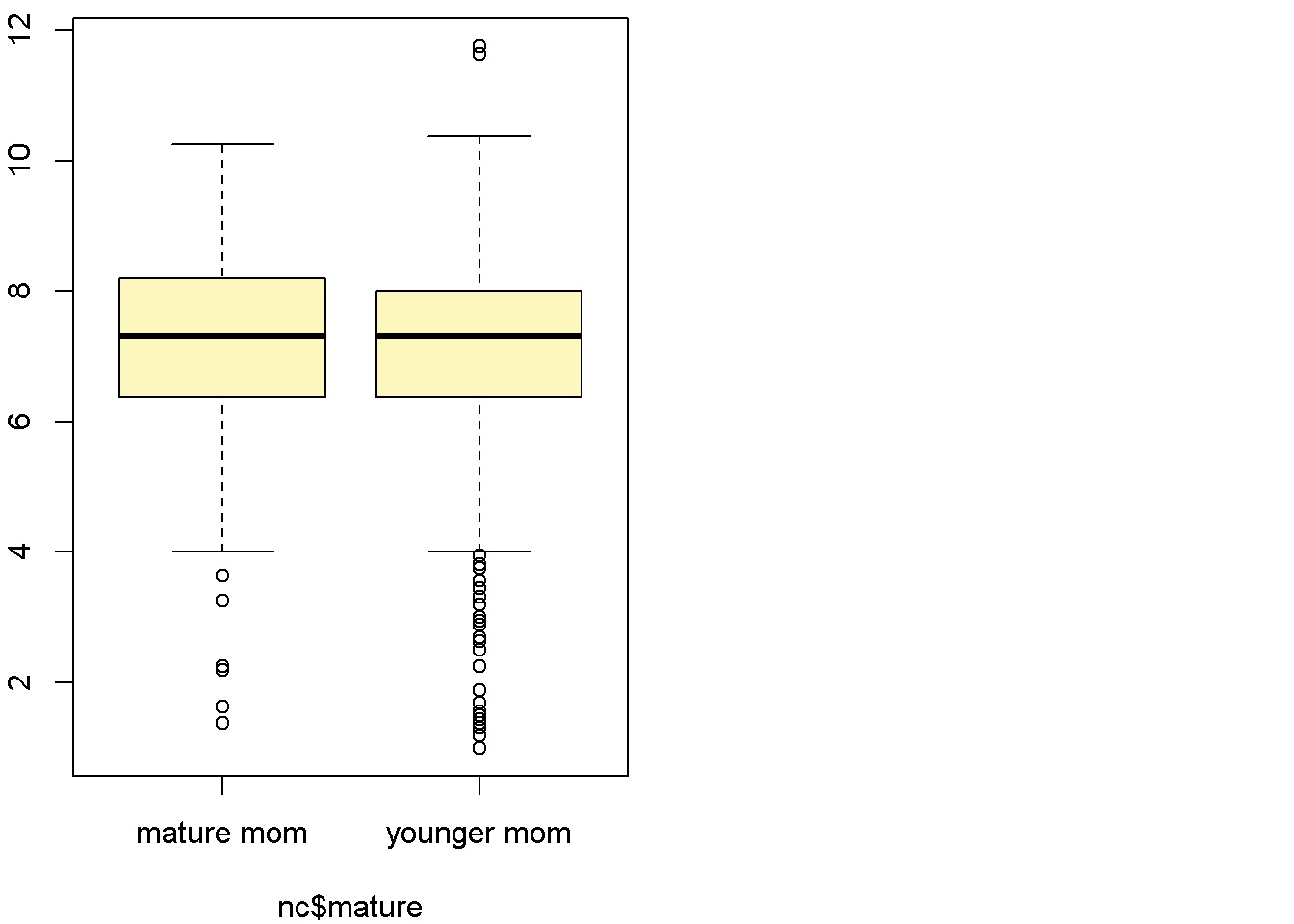## Observed difference between means (mature mom-younger mom) = 0.0283
##
## Standard error = 0.1525
## 95 % Confidence interval = ( -0.2705 , 0.3271 )

#### We are 95% confidence that there is no mean difference in weight gained during pregnancy between younger mothers and mature mothers because the 95% confidence interval (-0.2705, 0.3271) included zero.

###3.Now, a non-inference task: Determine the age cutoff for younger and mature mothers. Use a method of your choice, and explain how your method works.

max(nc$mage[nc$mature=="younger mom"])
##  34
min(nc$mage[nc$mature=="mature mom"])
##  35

#### The code is to look at mother’s age by category between younger mother and mature mother. The highest age in younger mother group is 34, and the lowest age in mature mother group is 35. So the cutoff for younger mother is before 35 years old and mother older than 35 years old is consider mature.

###5.Pick a pair of numerical and categorical variables and come up with a research question evaluating the relationship between these variables. Formulate the question in a way that it can be answered using a hypothesis test and/or a confidence interval. Answer your question using the inference function, report the statistical results, and also provide an explanation in plain language. #### Numerical pair: number of weeks of pregnancy and marital status #### Null hypothesis: There is no mean difference in weeks of pregnancy between married and unmarried mothers. Alternate Hypothesis: There is a mean difference in weeks of pregnancy between married and unmarried mothers

inference(y = nc$weeks, x = nc$marital, est = "mean", type = "ci", null = 0,
alternative = "twosided", method = "theoretical") 
## Response variable: numerical, Explanatory variable: categorical
## Difference between two means
## Summary statistics:
## n_married = 386, mean_married = 38.0803, sd_married = 3.4243
## n_not married = 612, mean_not married = 38.4951, sd_not married = 2.5628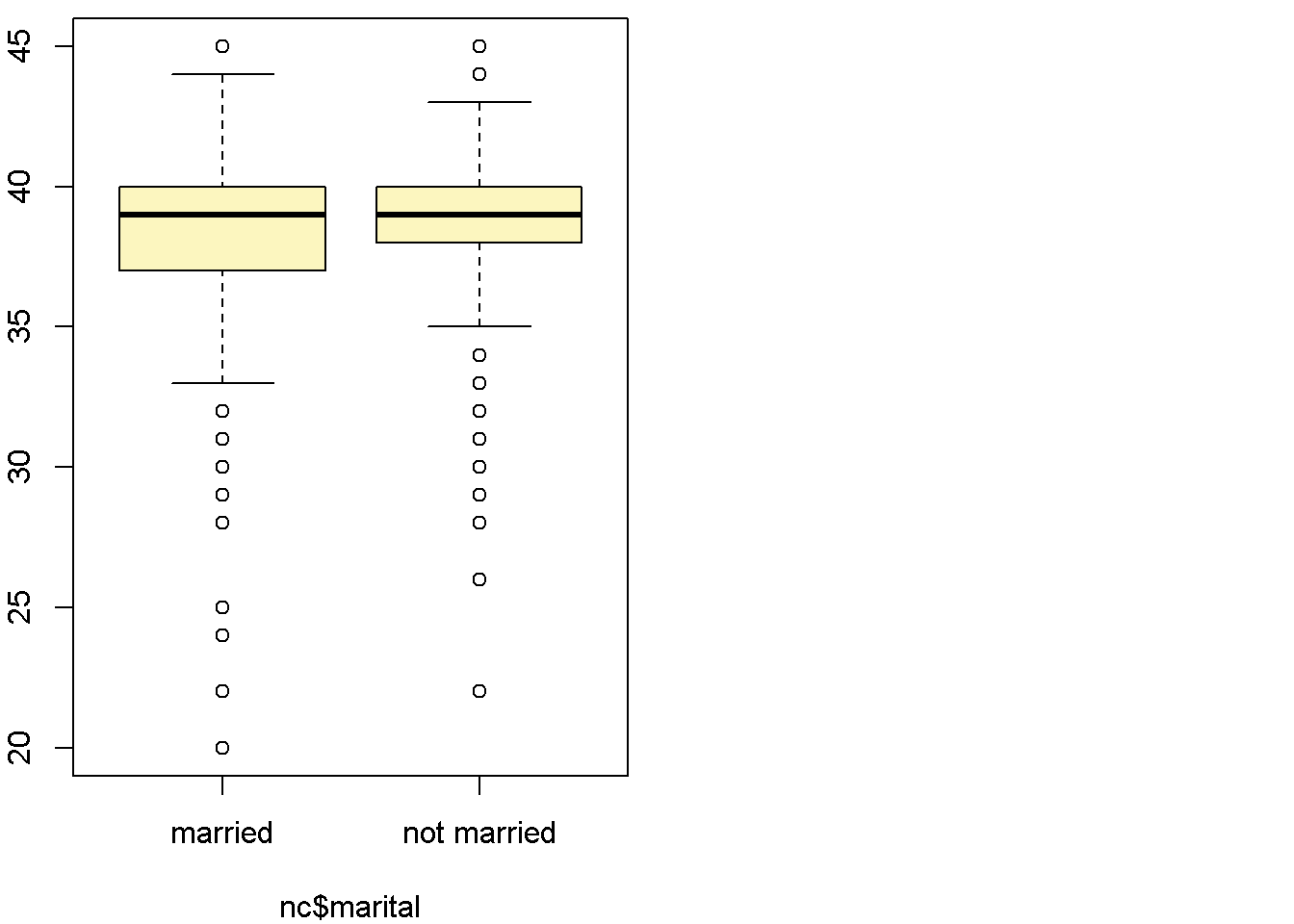## Observed difference between means (married-not married) = -0.4148
##
## Standard error = 0.2028
## 95 % Confidence interval = ( -0.8122 , -0.0174 )

Based on the hypothesis test, We are 95% confident that the true population mean for weeks of pregnancy in married women is less than that of not married mothers.